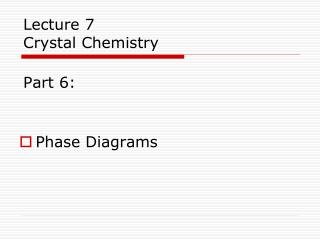DownloadDownload PresentationLecture 7 Crystal Chemistry Part 6:

# Lecture 7 Crystal Chemistry Part 6:

Télécharger la présentation## Lecture 7 Crystal Chemistry Part 6:

- - - - - - - - - - - - - - - - - - - - - - - - - - - E N D - - - - - - - - - - - - - - - - - - - - - - - - - - -
##### Presentation Transcript

1. Lecture 7Crystal ChemistryPart 6: • Phase Diagrams

2. We were discussing: Gibbs Free Energy • G – the energy of a system in excess of its internal energy. • This is the energy necessary for a reaction to proceed • Last time: G = E + PV - TS dG = VdP – SdT at constant T (δG/δP)T = V at constant P (δG/δT)P = -S Stable phases have the lowest G

3. Clapeyron Equation • Defines the state of equilibrium between reactants and product in terms of S and V dP/dT = ΔS / ΔV The slope of the equilibrium curve will be positive if S and V both decrease or increase with increased T and P

4. Vice > Vliquidso-ΔV Sice < Sliquidso+ΔS Ice to liquid V decr. and S incr. Slope of Phase Reaction Boundaries dP/dT = ΔS / ΔV so the line has a negative slope so the line has a negative slope Liquid to vapor V incr and S incr. Vliquid < Vvaporso +ΔV Sliquid < Svaporso +ΔS so the line has a positive slope Slope of curve positive if S and V both decrease or increase with increased T and P

5. Variables • Extensive Variables – dependent on the amount of material present • mass • volume • moles of atoms • Intensive Variables – independent on the amount of material present • pressure • temperature • density • compositional proportions

6. Gibbs Phase Rule rewritten F = C – Φ + 2 F – number of degrees of freedom of intensive variables (p, t, x) that will still preserve chemical equilibrium C – number of components (chemical entities) Φ – number of phases (minerals, volatiles)

7. One Component Phase Diagrams Illustrate Polymorphism Isochemical P & T are intensive variables Phase Rule: univariant lines F=1 C Al2SiO5 SiO2 CaCO3 F = C – Φ + 2 Kyanite = Sillimanite Φ = 2 C = 1 F = 1 – 2 + 2 = 1

8. Two Component Phase Diagrams Solid Solution Crystallization Eutectic Crystallization • Usually portrayed as isobaric (constant Pressure) T-X diagrams • For igneous systems, magma/melt is a phase of a simplified composition defined by the mineral phases of interest • Liquidus – denotes the temperature at which the liquid of a particular composition will begin to crystallize • Solidus denotes the temperature at which the liquid of a particular composition will be completely crystallized • A eutectic system has a single chemical composition that solidifies at a lower temperature than any other composition.

9. Eutectic Crystallization of 70% Anorthite (plag.) and 30% Diopside (px) Eutectic Point Lever Rule Proportions Anorthite Diopside Read Comments

10. At d, when the solids are the same composition as the original melt, Cooling stops until all of the liquid is used up. Then the solids cool. Solid Solution Crystallization Constant and complete interaction between solids and melt. Fe++ Mg++

11. Exsolution Textures Subsolidus Unmixing Pyroxene Hypersthene (Opx) exsolution lamellae in Augite (Cpx) host Alkali Feldspar Albite exsolution (perthite) in Microcline host

12. Multi-component Phase DiagramsIgneous Systems – Liquidus Diagrams Liquidus Surface Cotectic Lines Eutectic Point Cotectic: conditions where two or more solid phases crystallize at the same time, from a single liquid over a finite range of decreasing temperature.

13. We will do more of these with individual minerals, and will find them extremely useful in Petrology